DC power supply 12V 2A

Hi!
I want to design DC power supply 12V 2A. Here is what I have done.
Firstly, we need to transform AC main voltage. I will use transformer with ratio Np:Ns=16:1 :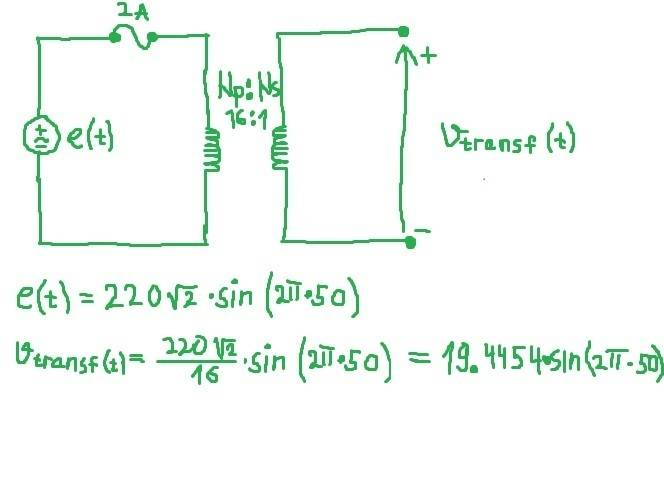We must choose diodes with maximum forward current more than 2 amperes. I will choose UG8JTHE3/45.. This diode has maximum forward current 8A and maximum reverse voltage 600V, Is=1.0025^(-12)A, Emission Coefficient n=1.74999 (according to Multisim model).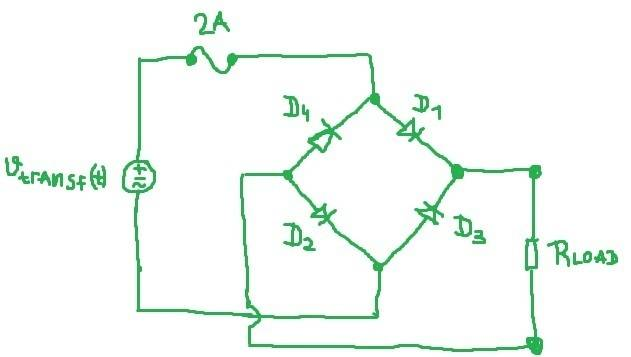Let's analyse circuit in case e(t)>0. I will not analyse case e(t)<0 since everything is same as in this case (only difference is of course that two other diodes conducts). In this case D1 and D2 are on, while D3 and D4 are off: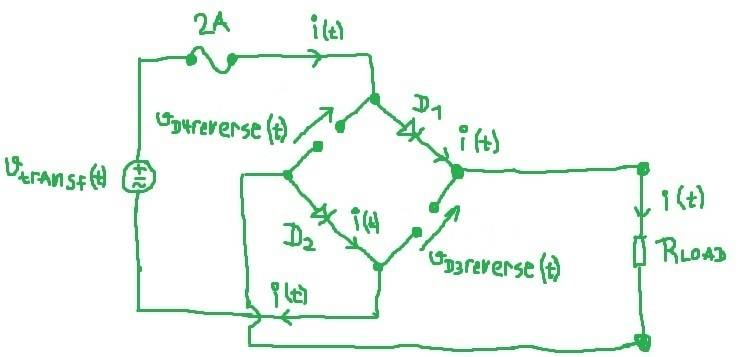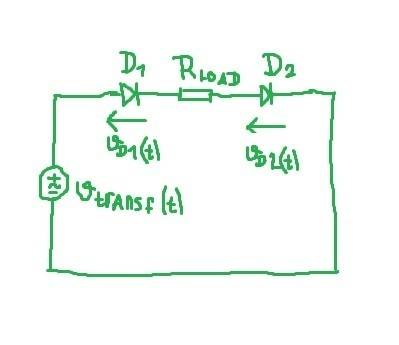Diodes D1 and D2 are same, same current flow through them so voltages across them are also same.
Since we want to calculate maximum (peak) value of voltage on diodes and peak value of power on diodes, peak value of power on transformer's secondary and lowest value of passive load we can connect on our power supply, we solve this circuit:
VD1max=VD2max=VDmax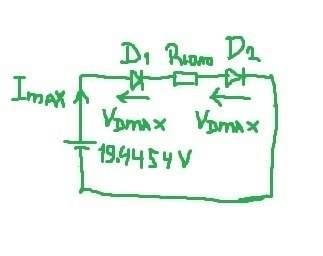$$I_m_a_x=I_s(e^{\frac{V_D_m_a_x}{nV_t}}-1)...(1)$$
$$V_D_m_a_x=\frac{1}{2}(19.4454-2R_l_o_a_d)...(2)$$
If we put (2) in (1) we get:
$$2=1.0025*10^{-12}(e^{\frac{19.4454-2R_l_o_a_d}{2*1.74999*0.02589}}-1)$$(Vt=0.0258 at temperature 27 degrees Celsius).
If we solve this nonlinear equation in Matlab, we get Rload=8.4395Ohms. This would be lowest passive load value we can connect to our supply. So when Rload=8.4395Ohms, peak value of current in circuit is Imax=2A. In that case, peak value of voltage across diodes will be VDmax=(1/2)*(19.4454-2*8.4395)=1.2832V. When voltage at transformer's output is at peak value 19.4454V and current is 2A, power that is dissipated is Ptransf.max=19.44542*2=38.8908W. For everything to work fine, we should choose some transformer with higher power rating than this. PIV (peak inverse voltage) on diodes is: PIV=Rload*Imax+VDmax=18.1622V<<600V.
Peak value of power on diodes is PD1max=PD2max=PD3max=PD4max=VDmax*Imax=1.2832V*2A=2.5664W.
Output voltage will pulsate from 0v to Imax*Rload=2A*8.4395Ohms=16.8790V.
My calculations match with Multisim. What do you think? What power rating should transformer have exactly? Maybe 50W?
It is necessary now to smooth pulsating voltage. What is best way to do this? Would it be enough to use just one capacitor and one voltage regulator?

Last edited:

I don't know why latex doesn't work...

jim hardy
Gold Member
Dearly Missed
•etf and davenn
Hi jim hardy, thanks for reply but It doesn't help me a lot :( I know that I have to put capacitance in parallel with my load but It will not be enough, right? There must be also some voltage regulator for smooth output voltage, as I know. Can someone go through my calculations above?

And here are 3 equations which Latex didn't show correctly: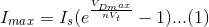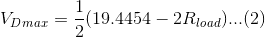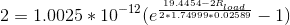jim hardy
Gold Member
Dearly Missed
It is necessary now to smooth pulsating voltage. What is best way to do this? Would it be enough to use just one capacitor and one voltage regulator?

you can approximate capacitor size by C = 2 amps /(dv/dt) , choose dv/dt to give however little ripple you want.regulators abound, here's a popular one.
pay attention to paragraph "external capacitors" on page 7.

Your fuse should protect the transformer's primary winding
2 amps at 220 volts is 440 VA which seems a lot for a 24 VA supply.
Are you oversizing your transformer by that much?

Lastly remember that current in transformer winding flows only during the brief charging intervals at voltage peaks.
So it's far from sinusoidal.
Meaning you get more voltage drop in the transformer winding than you'd expect, hence more voltage droop as load increases.which is why in that Hammond link, V(avg)DC is so much less than V(peak )DC.
Observe the benefit of choke input.

•etf